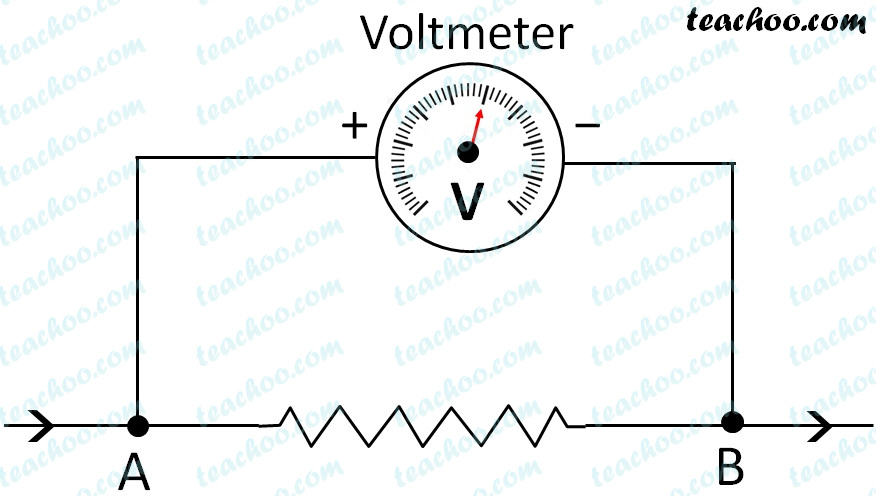Concepts

Class 10
Chapter 12 Class 10 - Electricity

## Does Water flow from one tank to another?

No

This is because there is no pressure

## What if we increase height of one tank. Does Water flow from one tank to another?

Yes

This is because water now flows from tank having higher pressure to tank having lower pressureHence, for flow of water, there should be difference in water pressure

Similarly for flow of Electricity, there should be difference in Electricity Pressure

This difference in Electric Pressure is called Potential Difference

## What is Potential Difference?

The potential difference between two points in an electric circuit

is defined as the work done to Move unit Electric Charge from one Point to another in an electric circuit.

Potential Difference = Work Done/Charge

V = W/Q

## How is Potential Difference Measured?

It is measured in Volt (V)

It is named after Italian Physicist Alessandro Volta

We Know that

Potential Difference = Work Done/Charge

Since Potential Difference is measured in Volt(V),Work Done in Joule (J) and Charge in coulomb(C).

We can say

1 Volt = 1 Joule/1 Coulomb

1 V = 1 J /1 C

Hence, 1 Volt is amount of potential difference produced when 1 Joule of Work is done to move 1 Coulomb of Charge from One point to another, in an electric circuit.

## How is Potential Difference Measured?

It is measured by Device called Voltmeter

It is always connected parallel to points where potential difference is to be measured

Potential difference between two points in an electric circuit can be created with the help of cell or battery.

Circuit diagram to show voltmeter## Questions

Q1 Page 202 - Name a device that helps to maintain a potential difference across a conductor.

Q2 Page 202 - What is meant by saying that the potential difference between two points is 1 V?

Q3 Page 202 - How much energy is given to each coulomb of charge passing through a 6 V battery?

Example 12.2 - How much work is done in moving a charge of 2 C across two points having a potential difference 12 V?

Learn in your speed, with individual attention - Teachoo Maths 1-on-1 Class